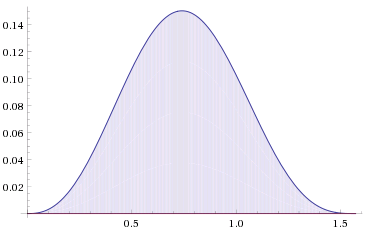# A Normal Distribution?

Calculus Level 4Given that the indefinite integral

$\large \int \sin^{\frac {5}{2}} x \cos^3 x \space \mathrm{d}x$

equals to

$\large 2 \sin^{ \frac {A}{2} } x \left [ \frac {1}{B} - \frac {1}{C} \sin^2 x \right ]$

neglecting the arbitrary constant.

Find the value of $A + B - C$.